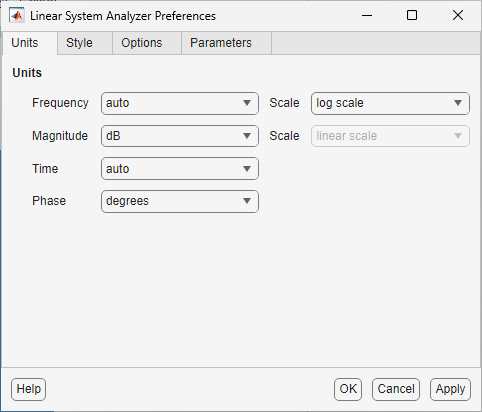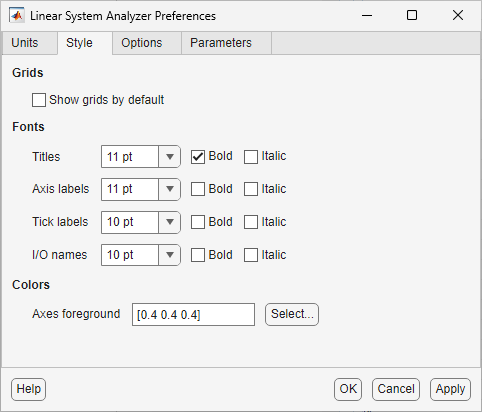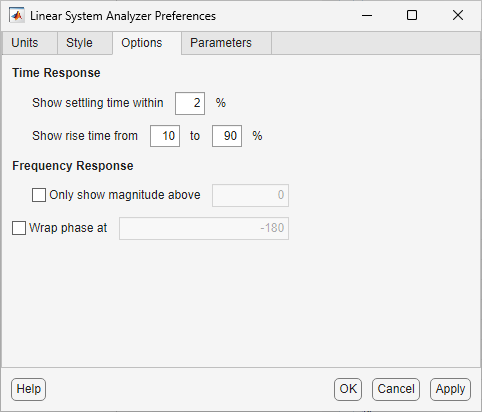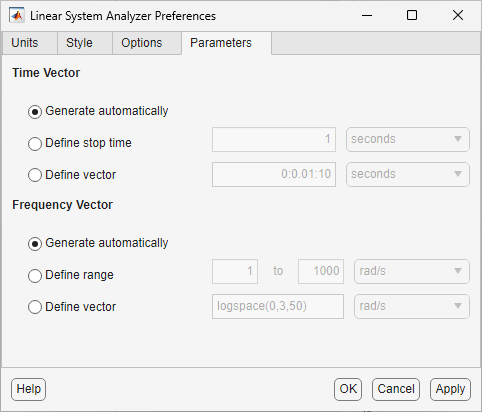## Linear System Analyzer Preferences Editor

### Opening the Linear System Analyzer Preference Editor

In the Linear System Analyzer, select Edit > Linear System Analyzer Preferences. The Linear System Analyzer Preferences dialog box let you customize various Linear System Analyzer properties, including units, fonts, and various other characteristics. This figure shows the editor open to its first pane.### Units PaneYou can select the following on the Units pane:

• Frequency

The default `auto` option uses `rad/TimeUnit` as the frequency units relative to the system time units, where `TimeUnit` is the system time units specified in the `TimeUnit` property of the system on frequency-domain plots. For multiple systems with different time units, the units of the first system is used.

For the frequency axis, you can select logarithmic or linear scales.

• Magnitude — Decibels (dB) or absolute value (abs)

• Phase — Degrees or radians

• Time

The default `auto` option uses the time units specified in the `TimeUnit` property of the system on the time- and frequency-domain plots. For multiple systems with different time units, the units of the first system is used.

### Style Pane

Use the Style pane to toggle grid visibility and set font preferences and axes foreground colors for all plots in the Linear System Analyzer. This figure shows the Style pane.You have the following choices:

• Grid — Activate grids for all plots in the Linear System Analyzer

• Fonts — Set the font size, weight (bold), and angle (italic). Select font sizes from the menus or type any font-size values in the fields.

• Colors — Specify the color vector to use for the axes foreground, which includes the X-Y axes, grid lines, and tick labels. Use a three-element vector to represent red, green, and blue (RGB) values. Vector element values can range from 0 to 1.

• If you do not want to specify the RGB values numerically, press the Select button to open the Select Colors window.

### Options Pane

The Options pane has selections for time responses and frequency responses.For time response plots, the following options are available:

• Show settling time within xx% — Set the threshold of the settling time calculation to any percentage from 0 to 100%. The default is 2%.

• Specify rise time from xx% to yy%— The standard definition of rise time is the time it takes the signal to go from 10% to 90% of the final value. Specify any percentages you like (from 0% to 100%), provided that the first value is smaller than the second.

For frequency response plots, the following options are available:

• Only show magnitude above — Specify a lower limit for magnitude values in response plots so that you can focus on a region of interest.

• Wrap phase — Wrap the phase into the interval [–180º,180º). To wrap accumulated phase at a different value, enter the value in the Branch field. For example, entering 0 causes the plot to wrap the phase into the interval [0º,360º).

### Parameters Pane

Use the Parameters pane, shown below, to specify input vectors for time and frequency simulation.The defaults are to generate time and frequency vectors for your plots automatically. You can, however, override the defaults as follows:

• Time Vector:

• Define stop time — Specify the final time value for your simulation

• Define vector — Specify the time vector manually using equal-sized time steps

• Frequency Vector:

• Define range — Specify the bandwidth of your response. Whether it's in rad/sec or Hz depends on the selection you made in the Units pane.

• Define vector — Specify the vector for your frequency values. Any real, positive, strictly monotonically increasing vector is valid.